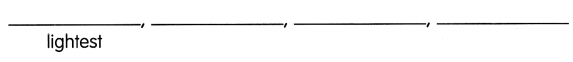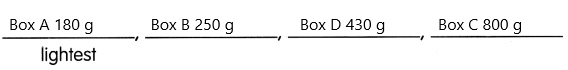# Math in Focus Grade 2 Chapter 8 Practice 4 Answer Key Comparing Masses in Grams

Practice the problems of Math in Focus Grade 2 Workbook Answer Key Chapter 8 Practice 4 Comparing Masses in Grams to score better marks in the exam.

## Math in Focus Grade 2 Chapter 8 Practice 4 Answer Key Comparing Masses in Grams

Find the mass of each vegetable. Then fill in the blanks.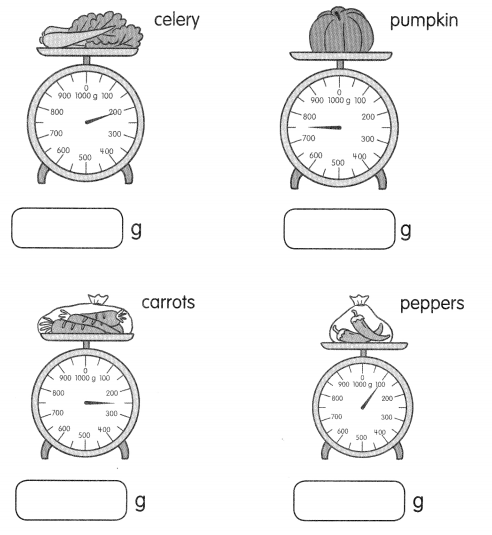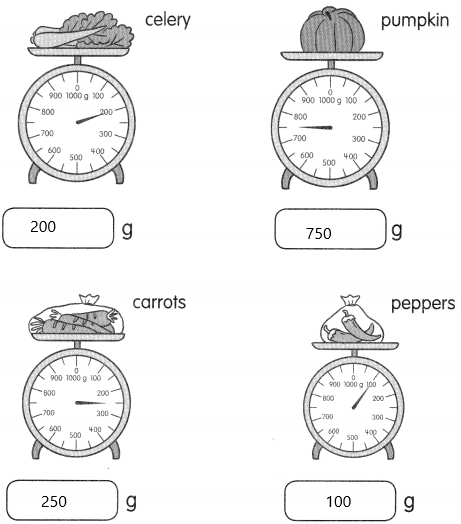Question 1.
The ___ is the heaviest.
The pumpkin is having 750 grams. So it is the heaviest.

Question 2.
The ___ are the lightest.
The mass of peppers is 100 grams which are the lightest.

Question 3.
The pumpkin is ____ grams heavier than the celery.
The mass of the pumpkin is 750 grams.
The mass of celery is 250 grams.
The number of more grams the pumpkin is heavier than celery=X
Subtract the mass of pumpkin and mass of celery.
X=750-250
X=500
Therefore, 500 grams more than celery.

Question 4.
The ___ is heavier than the bag of peppers but lighter than the bag of carrots.
The mass of peppers=100 grams.
The mass of carrots=250 grams.
we need to calculate the thing which is heavier than peppers and lighter than carrots.
Now observe the masses of vegetables. Compare all the vegetables to each other.
The mass of celery is 200 grams.
It is the only one which is lighter than carrots and heavier than peppers.

Look at the boxes. Then fill in the blanks.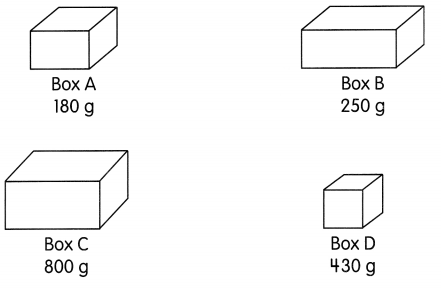Question 5.
Which is the heaviest? Box ____
Explanation:
The mass of box A is 180 g
The mass of box B is 250 g
The mass of box C is 800 g
The mass of box D is 430 g
Now observe which is having the highest mass.
The heaviest box is 800 grams which are box C.

Question 6.
Which is the lightest? Box ___
Explanation:
The mass of box A is 180 g
The mass of box B is 250 g
The mass of box C is 800 g
The mass of box D is 430 g
Now observe which is having the lightest mass.
The lightest box is 180 grams which are a box A.

Write heavier or lighter.

Question 7.
Box B is _____________ than Box D.
Explanation:
The mass of box B is 250 g
The mass of box D is 430 g
Box B is lesser than box D.
Therefore, box B is lighter than box D.

Question 8.
Box D is ____________ than Box A.
Explanation:
The mass of box D is 430 g
The mass of box A is 180 g
Box D is greater than box A.
Therefore, box D is heavier than box A.

Fill in the blanks.

Question 9.
Box C is ___ grams heavier than Box B.
The mass of box B is 250 g
The mass of box C is 800 g
To calculate the number of more grams heavier than box B. Assume it as X.
X=800-250
X=550
Therefore, Box C is 550 grams heavier than Box B.

Question 10.
Box A is ___ grams lighter than Box C.
Explanation:
The mass of box A is 180 g
The mass of box C is 800 g
To calculate Box A is how much lighter than Box C. Assume it as X
X=800-180
X=620
therefore, Box A is 620 grams lighter than Box C.

Question 11.
Order the boxes from the lightest to the heaviest.